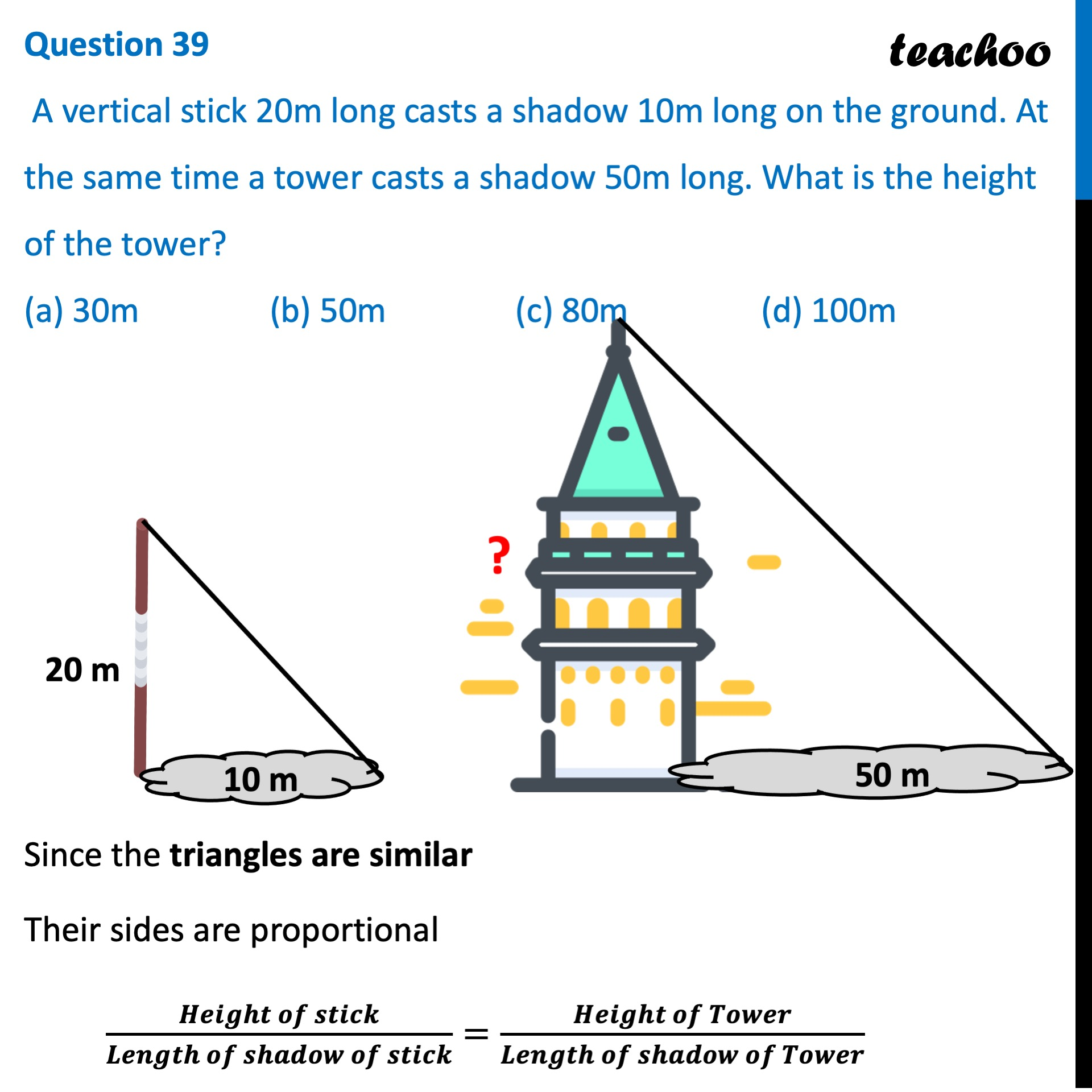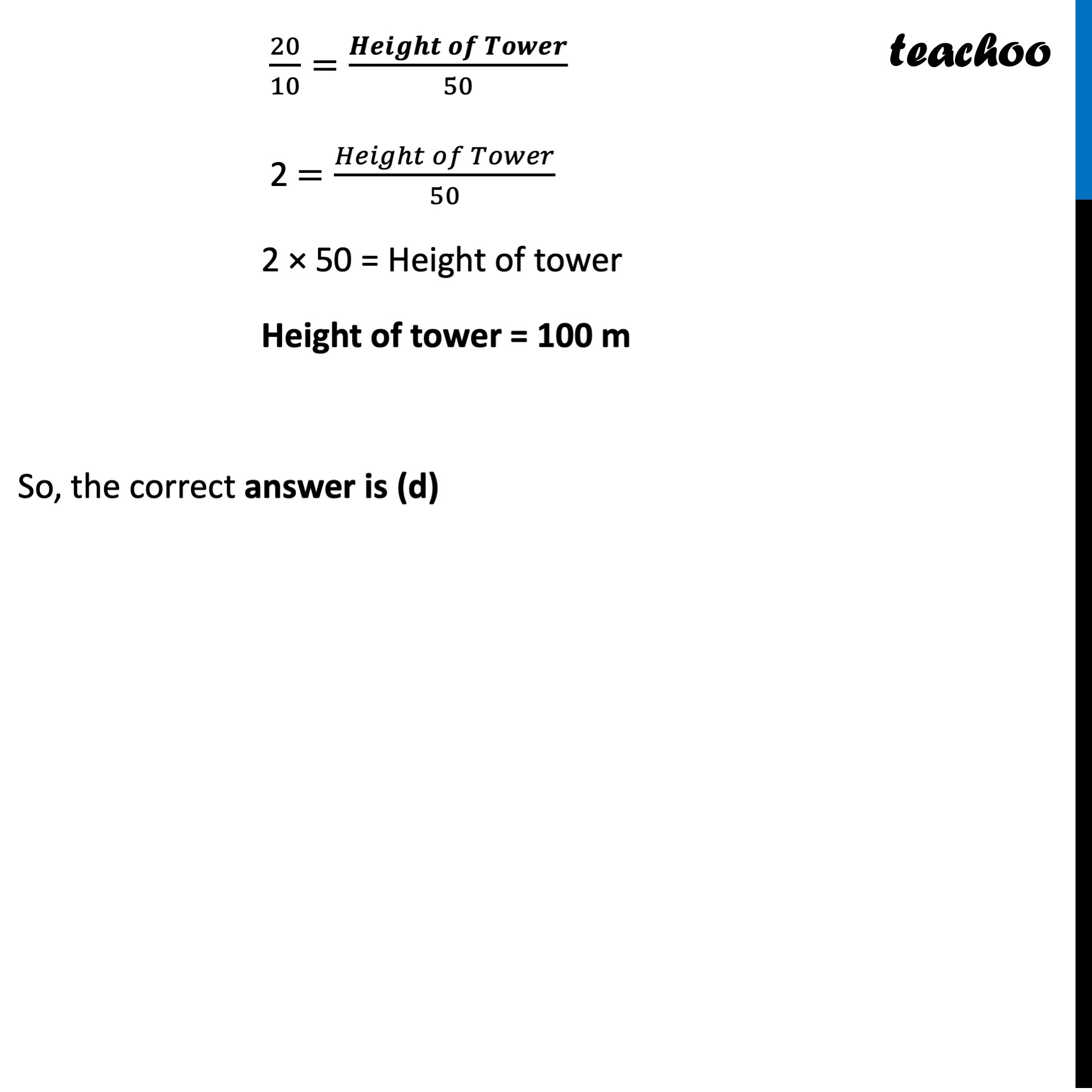## (a) 30m   (b) 50m   (c) 80m   (d) 100m1. Class 10
2. Solutions of Sample Papers for Class 10 Boards
3. CBSE Class 10 Sample Paper for 2022 Boards - Maths Basic [MCQ]

Transcript

Question 39 A vertical stick 20m long casts a shadow 10m long on the ground. At the same time a tower casts a shadow 50m long. What is the height of the tower? (a) 30m (b) 50m (c) 80m (d) 100m Since the triangles are similar Their sides are proportional (𝑯𝒆𝒊𝒈𝒉𝒕 𝒐𝒇 𝒔𝒕𝒊𝒄𝒌)/(𝑳𝒆𝒏𝒈𝒕𝒉 𝒐𝒇 𝒔𝒉𝒂𝒅𝒐𝒘 𝒐𝒇 𝒔𝒕𝒊𝒄𝒌)=(𝑯𝒆𝒊𝒈𝒉𝒕 𝒐𝒇 𝑻𝒐𝒘𝒆𝒓)/(𝑳𝒆𝒏𝒈𝒕𝒉 𝒐𝒇 𝒔𝒉𝒂𝒅𝒐𝒘 𝒐𝒇 𝑻𝒐𝒘𝒆𝒓) 20/10=(𝑯𝒆𝒊𝒈𝒉𝒕 𝒐𝒇 𝑻𝒐𝒘𝒆𝒓)/50 2 =(𝐻𝑒𝑖𝑔ℎ𝑡 𝑜𝑓 𝑇𝑜𝑤𝑒𝑟)/50 2 × 50 = Height of tower Height of tower = 100 m So, the correct answer is (d)